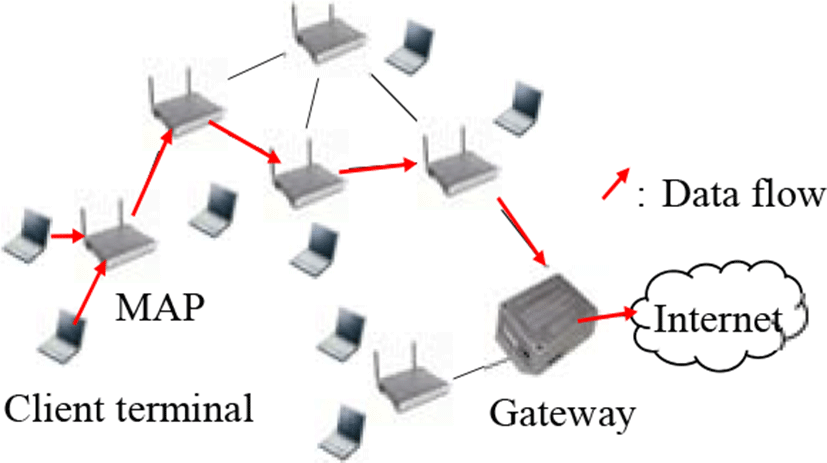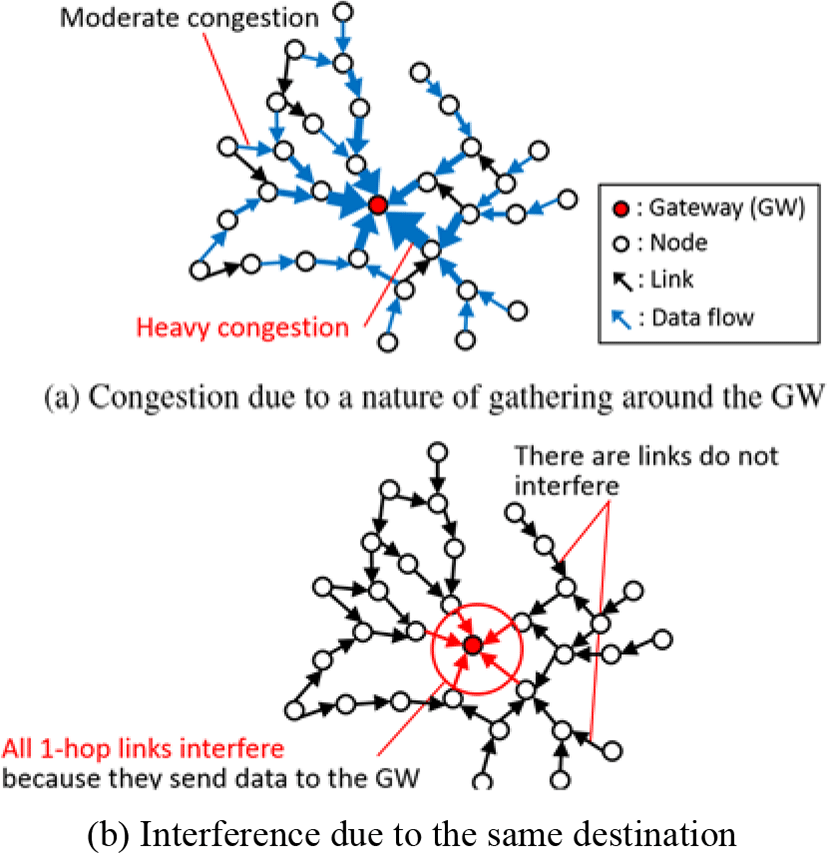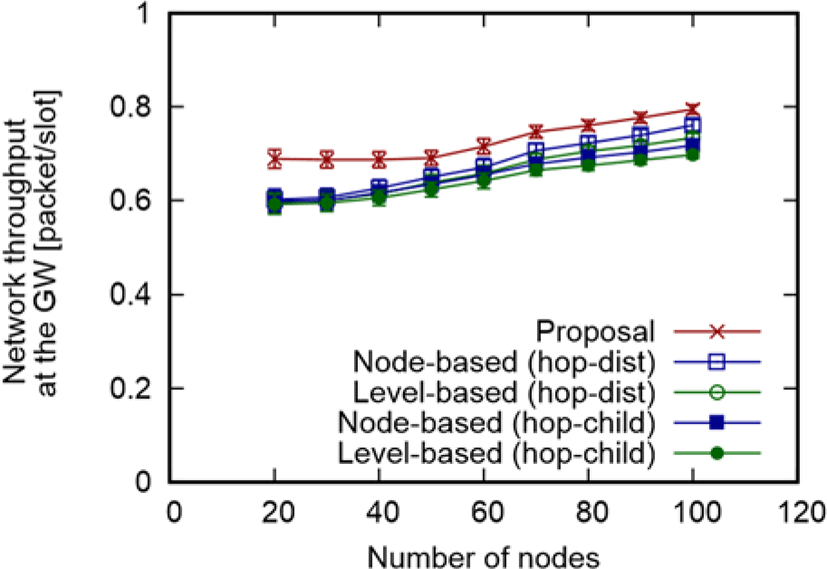Journal of Multimedia Information System
Korea Multimedia Society
Section C

# A Divided Scheduling Method based on Structural Characteristics in Wireless

Yuriko Yoshino1,*, Masafumi Hashimoto1, Naoki Wakamiya1
1Graduate School of Information Science and Technology, Osaka University, Osaka, Japan, y-yosino@ist.osaka-u.ac.jp,m-hasimt@ist.osaka-u.ac.jp, wakamiya@ist.osaka-u.ac.jp.
*Corresponding Author: Yuriko Yoshino, Graduate School of Information Science and Technology, Osaka University, 1-5 Yamadaoka, Suita, Osaka 565-0871, Japan, y-yosino@ist.osaka-u.ac.jp.

© Copyright 2016 Korea Multimedia Society. This is an Open-Access article distributed under the terms of the Creative Commons Attribution Non-Commercial License (http://creativecommons.org/licenses/by-nc/4.0/) which permits unrestricted non-commercial use, distribution, and reproduction in any medium, provided the original work is properly cited.

Received: Dec 18, 2016 ; Revised: Dec 30, 2016 ; Accepted: Dec 31, 2016

Published Online: Dec 31, 2016

## Abstract

Wireless mesh networks (WMNs) are used for metropolitan area network that requires high network throughput for handling many users. TDMA-based access is a common solution for this problem and several scheduling methods have been proposed. However, existing heuristic methods have room for improvement at network throughput although they are low complexity. In this paper, we propose a novel divided scheduling method based on structural characteristics in order to improve network throughput in WMNs. It separately schedules neighbor links of gateways and that of the other links by different scheduling algorithms. Simulation-based evaluations show that our proposal improves up to 14% of network throughput compared with conventional scheduling algorithm script.

Keywords: Wireless; Divided scheduling; Structural characteristic; GWN; WMN

## I. INTRODUCTION

Wireless mesh networks (WMNs) are promising technologies as the solution for metropolitan area networks, providing last few miles connectivity . Figure 1 represents a typical example of WMNs. The WMN consists three components: a gateway (GW), mesh access points (MAPs), and client terminals. Client terminals associate to nearby MAPs to access node in the external network such as the Internet. MAPs are mesh routers with an access point function. They relay traffic generated from terminals by multi-hop to a GW that connects to the external networks. In what follows, we regard traffic from client terminals as that from the MAP with which they associate. Unless otherwise noted, MAPs are called nodes in this paper.Fig 1. An example of WMNs

WMNs for metropolitan area networks require large network capacity since they handle many user requests. As a result, network throughput is a primary concern. TDMA-based access is a common way to improve network throughput. Therefore, many scheduling methods have been proposed .

Li et al. in  formulated the combinatorial optimization for throughput and energy efficiency for WMNs. However, it can only be applied to small networks that consist of few nodes because this problem is NP complete. In , Gunasekaran et al. proposed near-optimal solution based on Genetic

Algorithm (GA) for the combinatorial optimization problem to maximize throughput and channel utilization. However, it has some difficulty in setting parameters according to various situations. One of solutions for the issue is to use a heuristic method with simple parameter settings.

In order to realize simplicity, Liu et al. in  proposed centralized scheduling algorithms that determine combination schedule of upload and download traffic based on characteristics of congestion caused by each traffic flow. In , Ergen and Varaiya also proposed centralized scheduling algorithms, which first make sets of nodes based on interference relationships among nodes and then assigns them to time slots in order. They are low complexity since they use simple metrics such as hop counts and interference relationship among nodes. However, they have room for improvement at network throughput. It is because combinations of various factor, including topology, traffic demand, and interference, cause the throughput degradation. Therefore, we need to further improve network throughput for increase in user clients by focusing on them.

To this end, in this paper, we propose an effective heuristic algorithm for the scheduling problem in WMNs, which exploits interference in terms of the structural characteristics. Based on this characteristics, we divide the scheduling into GW-neighbor (GWN) scheduling and non-GW-neighbor (nGWN) scheduling. GWN scheduling assigns links to time slots by focusing on interference between two-hop links. After that, in nGWN scheduling, we assign the remain links to time slots in order to integrate with the GWN schedule.

The rest of this paper is organized as follows. Section 2 first introduces the structural characteristics of WMNs. In Section 3, we then describe the proposed method in details. In Section 4, we assess the performance of our proposal through simulation experiments. Finally, we conclude the paper and present the future work in Section 5.

## II. Structural characteristics of WMNs

Figure 2 depicts a WMN where a single GW is located at the center of the area. We assume upstream traffic are generated from each node in the WMNs. Each node sends data toward the GW. When nodes do not have one-hop links to the GW, they forward data to their parent nodes. On receiving data, nodes forward then to their parent nodes until data reaches the GW.Fig. 2. Structural characteristics of WMNs

Figure 2(a) shows behavior of gathering data at the GW. All data are gathered around the GW because all nodes send data toward the GW. As a result, links around the GW are more congested than those not around the GW.

Furthermore, as shown in Figure 2(b), all one-hop links interfere because they send data to the same destination, i.e., the GW. On the contrary, the other links do not always interfere due to their different parent nodes.

## III. Proposed scheduling method

Due to the characteristics shown in Section 2, to maximize throughput, links around the GW need strict scheduling, whereas the other links may be scheduled loosely compared with them. Therefore, we divide the scheduling into the scheduling of links around the GW and the scheduling of links not around the GW.

The proposed method first assigns links around the GW to time slots, called as GWN scheduling. Specifically, based on interference among two-hop links, the GWN scheduling assigns one-and two-hop links from the GW to time slots under the assumption that all two-hop nodes have data corresponding to the amount of data their all descendant nodes have. After that, the proposed method assigns the remain links, i.e., links that are far from the GW more than three-hop, to time slots, called as nGWN scheduling. The nGWN scheduling assigns the remain links to time slots in time for the schedule of two-hop links determined by the GWN scheduling.

3.1. GWN scheduling
3.1.1. Approach

Maximizing the throughput means minimizing the frame size that is the total number of time slots required to forward all data to the GW. Thus, to short the frame size, links that do not interfere should be assigned to the same time slot as far as possible. Unfortunately, one-hop nodes cannot be assigned to the same time slot because they interfere. Thus, we assign a link set that consists of a single one-hop link and multiple two-hop links that do not interfere with each other to a time slot consecutively. This implies that we need to decrease the number of time slots where a set of only two-hop links is assigned.

To this end, we introduce the residual transmission cost for effective choosing two-hop links which are assigned to the same slots with a one-hop link. The residual transmission cost indicates the residual number of time slots where an only two-hop link set is assigned until the end of scheduling. Therefore, it is better to assign the two-hop links whose residual transmission cost is largest to the same slot with a one-hop link.

In addition to this, we introduce the sub-schedules for losing the constraints in the nGWN scheduling. In the sub-schedule, sets of links are assigned to time slots based on the residual data of one-hop nodes at the beginning of this sub-schedule, which the number of data that they received at the previous sub-schedule. By doing so, in the sub-schedule, there is no dependency among links on the data transfer order, which means that time slots can be exchanged in the sub-schedule.

3.1.2. Algorithm overview

3.1.3. Residual transmission cost

In the following, we calculate the residual transmission cost ci for two-hop links that do not interfere with all one-hop links, whose set is denoted by E2f. Let E2c be a set of two-hop links that interfere with all one-hop links. Obviously, E2f = E2E2c where E2 is a set of two-hop links.

For calculating ci(iE2f), we utilize the set S(i) of sets of links in E2c ∪ { i } that do not interfere, whose elements are not subset of the other elements. S(i) is obtained as

$S\left(i\right)\text{\hspace{0.17em}}=\text{\hspace{0.17em}}\left\{S|S\text{\hspace{0.17em}}\subset \text{\hspace{0.17em}}{𝔓}_{C}\left(i\right),\text{\hspace{0.17em}}S\text{\hspace{0.17em}}\not\subset \text{\hspace{0.17em}}\forall \text{\hspace{0.17em}}P\in \text{\hspace{0.17em}}{𝔓}_{C}\left(i\right)\right\}$
(1)
${𝔓}_{C}=\left\{P|P\in 𝔓\left({E}_{2c}\text{\hspace{0.17em}}\cup \text{\hspace{0.17em}}\left\{i\right\}\text{\hspace{0.17em}}\right),\text{\hspace{0.17em}}\forall \text{\hspace{0.17em}}P,q\in P,p\text{\hspace{0.17em}}\ne \text{\hspace{0.17em}}q\wedge \text{\hspace{0.17em}}\left(p,q\right)\text{\hspace{0.17em}}\notin \text{\hspace{0.17em}}C\right\}$
(2)

where 𝔓(⋅) is a power set, C is a set of interference relationships between two links, and 𝔓C(i) is a set of links that do not interfere among sets in a power set 𝔓(E2c ∪ {i}).

Links in E2c must be assigned to time slots with only two-hop links. Therefore, the number of time slots that include only two-hop links does not increase as far as iE2f is assigned to the same time slots with links in E2c, which reduces ci. For this, we need to find the number of minimum times n(S) that a link set S in S(i) is assigned to time slots, whose summation is equal to ci. Thus, we finally calculate ci as

$\mathrm{min}\text{\hspace{0.17em}}\sum _{S\in S\left(i\right)}\underset{j\in \text{\hspace{0.17em}}S}{\mathrm{max}}\text{\hspace{0.17em}}n\left(j,S\right)\text{\hspace{0.17em}}$
(3)
$\sum _{S\in S\left(i\right)}\sum _{\text{\hspace{0.17em}}j\in \text{\hspace{0.17em}}S}\text{\hspace{0.17em}}n\left(j,S\right)\text{\hspace{0.17em}}={R}_{d}\left(i\right)$
(4)

where n(j,S) is the number of times that j assigned as an element of S, whose maximum is equal to n(S), and Rd (i) is the total residual data of sending nodes of links in E2c ∪ {i}.

3.2. nGWN scheduling

After the GWN scheduling, the nGWN scheduling assigns the remain links to time slots to meet the constraint that two-hop nodes must have at least one data at the time, i.e., the deadline, scheduled by the GWN scheduling. Note that it does not matter from where data is if two-hop nodes can transmit according to the GWN schedule.

The nGWN scheduling determines the schedule SR, i.e., a set of link sets, whose element SR (k) is assigned to the k-th time slot. SR (k) only includes conflict-free links. Note that links in SR (k) do not interfere with one- and two-hop links assigned to k-th time slot. We need to find SR to minimize the frame size. To achieve this, given a set ER (k) of the remain links that have data at k-th time slot, we construct conflict-free link set from links in ER (k) in order of closest deadlines. ER (k) becomes empty if all remain links are scheduled. SR is finally obtained as

$\text{find:}{S}_{R}\text{\hspace{0.17em}}=\text{\hspace{0.17em}}\left\{{S}_{R}\left(k\right)\right\},\text{\hspace{0.17em}}k\text{\hspace{0.17em}}\ge \text{\hspace{0.17em}}1$
(5)
$\text{subject to:}{S}_{R}\left(k\right)\text{\hspace{0.17em}}\subseteq \text{\hspace{0.17em}}{E}_{R}\text{\hspace{0.17em}}\left(k\right)$
(6)

SR (k) is also calculated as

$\text{find:}{S}_{R}\left(k\right)\text{\hspace{0.17em}}=\text{\hspace{0.17em}}\left\{el\right\}$
(7)
$\text{subject to:}\exists e\text{\hspace{0.17em}}\in \text{\hspace{0.17em}}{S}_{R}\left(k\right),\text{\hspace{0.17em}}\forall \text{\hspace{0.17em}}i\text{\hspace{0.17em}}\in {E}_{R}\text{\hspace{0.17em}}\left(k\right),\text{\hspace{0.17em}}d\left(e,k\right)\text{\hspace{0.17em}}\le \text{\hspace{0.17em}}d\left(i,k\right)$
(8)
$d\left({e}_{l},k\right)\text{\hspace{0.17em}}\le \text{\hspace{0.17em}}d\left({e}_{l+1},k\right)$
(9)
$\forall i\text{\hspace{0.17em}}\in \text{\hspace{0.17em}}{E}_{RC}\left(k,l\right),\text{\hspace{0.17em}}d\left({e}_{l},k\right)\text{\hspace{0.17em}}\le \text{\hspace{0.17em}}d\left(i,k\right)$
(10)

where d(i,k) is the time slot number of the closest deadline of data that i has at k-th time slot for the two-hop node whose descendants include i, and ERC (k,l) is a set of candidates of l-th link for SR (k), i.e., ERC (k,l)= { i|iER (k),(em, j)∈ C, 1≤ ml − 1}.

Unfortunately, there are data that violate the deadline depending on the GWN schedule. When such a situation occurs, we insert time slots for the remain links to the GWN schedule until meeting the deadline.

## IV. Evaluation

4.1. Simulation settings

We evaluate our proposal by a hand-made C++ simulator. In the simulation, nodes are placed uniform-randomly within a square area of which a single GW is located at the center. Nodes have a single data toward the GW. Communication and interference among nodes follow the protocol model . In this model, each node can communicate with one within the transmission range, denoted by ru, and interferes with one within the interference range, denoted by ri. The simulation parameters are summarized in Table 1.

Table 1. Simulation parameters
Parameter Value
The number of nodes 20 - 100
The size of simulation area 200 × 200
Transmission range ru 40
Interference range ri 60

In the above settings, we compare our proposal with existing scheduling methods in : node-based and levelbased scheduling. They require a routing tree whose root is the GW as the input information. Thus, we give the two types of routing trees to them: Hop-dist and hop-child routing trees. In the hop-dist routing tree, we select a closest node as a parent from among nodes at minimum hop count from the GW. In the hop-child routing tree, we select a node whose children already have are fewest as a parent from among nodes at minimum hop count from the GW. Note that our proposal requires only the network topology.

4.2. Results

Figure 3 shows the average throughput at the GW varying the number of nodes which are obtained from results of 100 topologies with a 95% confidence interval.Fig. 3. The average throughput at the GW varying the number of nodes

Figure 3 reveals that our proposal improves up to 14.1% and 12.6% compared with level-based (hop-child) and node-based (hop-dist), respectively, when the number of nodes is 20. This is because our proposal can decrease slots that two-hop links transmit by using the residual transmission cost. As the number of nodes increases, all methods increase the throughput because the increase in the frame size is suppressed compared to the increase in the total traffic demand. We explain the reason of this at later by using Figure 4. Note that our proposal achieves the best throughput regardless of the number of nodes.Fig. 4. Frame size

In order to further understand, we present the frame size in Figure 4, corresponding to Figure 3. From Figure 4, as the number of nodes increase, the frame size in our proposal grows gently, whereas that in the other methods, excluding node-based with hop-dist, grow sharply. The reason of this is our proposal and node-based with hop-dist can use links which have less interference. In addition to this, frame size of all methods grows more sharply between the number of nodes is 20 from 50 than over 60. This is because hop counts of the route to the GW decreases over 60 nodes as the density of nodes increases. Therefore, the increase in the frame size is suppressed.

Although we omit results for biased demand situation due to space limitation, we confirmed that our proposal has same degree of improvement on the throughput.

## V. Conclusion

In this paper, we proposed the divided scheduling method based on structural characteristics of WMNs. The proposed method divides the scheduling into GWN scheduling and nGWN scheduling by the degree of interference. In GWN scheduling, we exploited the residual transmission cost for two-hop links in order to decrease time slots only two-hop links assigned. Through the simulation experiments, we showed that our proposal improves up to 14% compared with existing methods.

As future work, we plan to extend the proposed method to handle traffic fluctuations and node failures

## REFERENCES

.

P. H. Pathak and R. Dutta, “A Survey of Network Design Problems and Joint Design Approaches in Wireless Mesh Networks,” IEEE Communications Surveys & Tutorials, vol. 13, pp. 396–428, Sept. 2011..

M. Li, H. Nishiyama, Y. Owada, and K. Hamaguchi, “On energy efficient scheduling and load distribution based on renewable energy for wireless mesh network in disaster area,” in Proceeings of 2014 IEEE 13th International Conference on Trust, Security and Privacy in Computing and Communications, pp. 465–472, Sept 2014..

R. Gunasekaran, S. Siddharth, P. Krishnaraj, M. Kalaiarasan, and V. R. Uthariaraj, “Efficient Algorithms to Solve Broadcast Scheduling Problem in WiMAX Mesh Networks,” Computer Communications, vol. 33, no. 11, pp. 1325 – 1333, 2010..

S. Liu, S. Feng, W. Ye, and H. Zhuang, “Slot Allocation Algorithms in Centralized Scheduling Scheme for IEEE 802.16 Based Wireless Mesh Networks,” Computer Communications, vol. 32, no. 5, pp. 943 – 953, 2009..

S. C. Ergen and P. Varaiya, “TDMA Scheduling Algorithms for Wireless Sensor Networks,” Wireless Networks, vol. 16, pp. 985–997, May 2010..

M. Alicherry, R. Bhatia, and L. E. Li, “Joint Channel Assignment and Routing for Throughput Optimization in Multiradio Wireless Mesh Networks,” IEEE Journal on Selected Areas in Communications, vol. 24, pp. 1960–1971, Nov 2006.